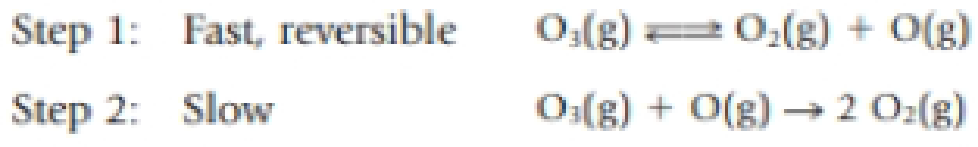# The ozone in the Earth’s ozone layer decomposes according to the equation 2 O 3 (g) → 3 O 2 (g) The mechanism of the reaction is thought to proceed through an initial fast equilibrium and a slow step: Show that the mechanism agrees with this experimental rate law: Rate = −(1/2)Δ[O 3 ]/Δ t = k [O 3 ] 2 [O 2 ].### Chemistry & Chemical Reactivity

9th Edition
John C. Kotz + 3 others
Publisher: Cengage Learning
ISBN: 9781133949640

#### Solutions

Chapter
Section### Chemistry & Chemical Reactivity

9th Edition
John C. Kotz + 3 others
Publisher: Cengage Learning
ISBN: 9781133949640
Chapter 14, Problem 67GQ
Textbook Problem
6 views

## The ozone in the Earth’s ozone layer decomposes according to the equation2 O3(g) → 3 O2(g)The mechanism of the reaction is thought to proceed through an initial fast equilibrium and a slow step:Show that the mechanism agrees with this experimental rate law:Rate = −(1/2)Δ[O3]/Δt = k[O3]2[O2].

Interpretation Introduction

Interpretation:

From the given set of mechanisms it should be proved that the given rate law matches with the given steps.

Concept introduction:

Rate law or rate equation: Rate law:

It is generally the rate equation that consists of the reaction rate with the concentration or the pressures of the reactants and constant parameters.

aA + bBxXRate of reaction = k [A]m[B]n

Order of a reaction:  The order of each reactant in a reaction is represented by the exponential term of the respective reactant present in the rate law and the overall order of the reaction is the sum of all the exponents of all reactants present in the chemical reaction. The order of the reaction is directly proportional to the concentration of the reactants.

Rate constant, k: The rate constant for a chemical reaction is the proportionality term in the chemical reaction rate law which gives the relationship between the rate and the concentration of the reactant present in the chemical reaction.

### Explanation of Solution

Examining the given set of steps it is clear that step 2 is the slowest step which means that it is the rate determining step and the rate equation for that is as follows,

Rate Law for Rate determing step,Rate = k[O3][O]FromStep1theKcvalueis as follows, Kc = [O2][O][O3][<

### Still sussing out bartleby?

Check out a sample textbook solution.

See a sample solution

#### The Solution to Your Study Problems

Bartleby provides explanations to thousands of textbook problems written by our experts, many with advanced degrees!

Get Started

Find more solutions based on key concepts
The FDA has extensive regulatory control over supplement sad. T F

Nutrition: Concepts and Controversies - Standalone book (MindTap Course List)

Where would you go on Earth to have a celestial pole at your zenith?

Horizons: Exploring the Universe (MindTap Course List)

What element makes up most of the detectable mass in the universe?

Oceanography: An Invitation To Marine Science, Loose-leaf Versin

Why are red dwarf star interiors thoroughly mixed?

Foundations of Astronomy (MindTap Course List)

An ice cube whose edges measure 20.0 mm is floating in a glass of ice-cold water, and one of the ice cube's fac...

Physics for Scientists and Engineers, Technology Update (No access codes included)